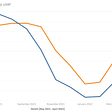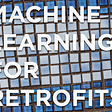# Does the Scaling of Data Matters in Prediction?

Most of the times we have a multiple feature with very medium to high magnitude or scaled values and this is obvious in the real-life data. For Example Annual Salary and the %of DA. Volume of Commodity and Exchange Rates.. and many more. Algorithms which are mostly dependent on the distance of the features which vary heavily in terms of scaling may not weigh all these in the same way. The parameter which has smaller values does not mean to be seen as less importance!!!

Hence, to make the predictions do not vary just because of the ‘X’ vars are of different scales, we can apply few techniques like Feature Scaling, Standardization or Normalization. Luckly, Scikit Learn has built-in functionalities which we will use. Well, I am not going to go into the details of Scikit-Learn and its functionalities, my main intentions is to show the Impact of un-scaled data use as ‘X’ vars!

Just One information I would really like to share is Scikit-Learn has MinMaxScaler and StandardScaler. In this episode I will be using StandardScaler for the scaling purpose.

I am straight heading to the code for the sake of more understanding.

`import pandas as pdimport seaborn as snsimport matplotlibimport matplotlib.pyplot as pltimport numpy as npimport warningsimport statsmodels.api as smfrom sklearn.linear_model import LinearRegressionfrom sklearn.model_selection import train_test_splitfrom sklearn import metricsfrom sklearn.metrics import mean_squared_errorfrom sklearn.metrics import r2_scorefrom sklearn.feature_selection import SelectKBestfrom sklearn.feature_selection import f_regressionfrom sklearn.feature_selection import RFEfrom sklearn.linear_model import RidgeCV, LassoCV, Ridge, Lassofrom yellowbrick.regressor import PredictionErrorfrom yellowbrick.regressor import ResidualsPlot%matplotlib inlinewarnings.filterwarnings('ignore')####################################### Loading the data####### Dataset has weekly data from 2015df = pd.read_csv ('02RxTXDataMv2.csv',parse_dates='Value_Date'])df.tail()`

INFO: Observe the data, we have RxT (y) Variable and rest are X vars in different scale

Now here is the correlation of all the ‘X’ vars with our RxT. Also I would be more interested to show only those vars which are having more then 50% of Correlation!!!

`corr = df.corr()cor_target = abs(corr['RxT'])#Selecting highly correlated featuresrelevant_features = cor_target[cor_target>0.5]relevant_features`

Please pay attention to our USEx var… it is 74.25% correlated with RxT. So this seems to be a good predictor…. hmmm lets build the model and see how it works out.

Analyse visually how our best correlated vars looks like

`xCorr = df2.corr()['RxT']df2RbCorr = pd.DataFrame({'Features':xCorr.index, 'CorrVal':xCorr.values})df2RbCorr = df2RbCorr.sort_values(by=['CorrVal'], ascending=False)plt.figure(figsize=(18,5))splot = sns.barplot(x = df2RbCorr['Features'], y=df2RbCorr['CorrVal'])for p in splot.patches: splot.annotate(format(p.get_height(), '.2f'),  (p.get_x() + p.get_width() / 2., p.get_height()),  ha = 'center', va = 'center',  xytext = (0, 9),  textcoords = 'offset points')plt.xticks(rotation=90)plt.show()`

Model building with values (No Scaling)

`df7x = df.copy()# Managing NaN if anydf7x.dropna(inplace=True)# Independent (Good) Vars and Target varx = df7x[['RxFC','USB', 'USEx','HSM']]y = df7x['RxT']# Training on 70% Testing 30%x_train, x_test, y_train, y_test = train_test_split(x, y, test_size=0.30, random_state=1 )lm4 = LinearRegression()lm4.fit(x_train, y_train)# Lets Predict the model with Testing Set yPred = lm4.predict(x_test)viz = PredictionError(lm4).fit(x_train, y_train)viz.score(x_test, y_test)viz.poof();`

I am using ‘yellowbrick’ package for visualization.. you can refer more here and a quickstart guide

Well, our model looks pretty good with 94.6% of r2!

Lets also see important metrics as well:

`print('MSE:',metrics.mean_squared_error(y_test,yPred))print('R²:',r2_score(y_test, yPred))print('MAE:',metrics.mean_absolute_error(y_test,yPred))print('MSR:',np.sqrt(metrics.mean_squared_error(y_test, yPred)))print('COF:',lm4.coef_)print('INT:',lm4.intercept_)`

Now, its time to do the predictions. I am passing 8 rows of X vars from the RxTSample.csv file. Please notice the RxT column, this is only for the ref as what should be predicted value when we pass the X vars to our model. Don't forget we have a 95.6% of r2_score and all the X Vars are more than 75% correlated.

`# Prepare Data UploaddfRxT = pd.read_csv('RxTSample.csv', parse_dates=['Value_Date'], index_col='Value_Date')dfRxT.dropna(inplace=True)dfRxT.head(10)`

Code for Forecasting (prediction):

`print('Value Date RxT_Actual Predicted_Value Variance')for index, row in dfRBT.iterrows(): # preparing X Vars to pass to Predict Fn paramsRB14D = [row['RxFC'], row['USB'], row['USEx'], row['HSM']]   # Calling Linear Regression predict with xVars prid = lm4.predict([paramsRB14D]) print(index.strftime('%d-%m-%Y'),' ',  "{:.1f}".format(row['RxT']),' ', list(map('{:.1f}'.format,prid)),' ', list(map('{:.1f}'.format,(prid — row['RxT']))))`

OOPs!!! WE have a BIG variance….. well, so far the data we used is un-scaled. We will now try to introduce the Scaled data and observe the code and prediction.

Here, I am showing the snippet of the code where I implemented the StandardScaler for the scaling purpose of our data.

`from sklearn.preprocessing import StandardScaler# Copy to temporary DataSet… its is always gooddfTmp = df.copy()# Deleting Date column otherwise # you will get 'invalid promotion error'dfTmp.drop('Value_Date', axis=1, inplace=True)dataScaler = StandardScaler()data = dataScaler.fit_transform(dfTmp)# Converting back to DataFramedf2 = pd.DataFrame(data)df2.rename(columns={0:'RxT',1:'RxFC', 2:'USB', 3:'USEx', 4:'HSM'}, inplace=True)# Copy our Scaled data into the modeldf7x = df2.copy()# Managing NaN if anydf7x.dropna(inplace=True) # Independent (Good) Varsx = df7x[['RxFC', 'USB', 'USEx', 'HSM']] y = df7x['RxT'] # Training on 70% Testing 30%x_train, x_test, y_train, y_test = train_test_split(x, y, test_size=0.30, random_state=1 ) lm4 = LinearRegression()lm4.fit(x_train, y_train)# Lets Predict the model with Testing Set yPred = lm4.predict(x_test)`

Showing the PredictionError with the best LM line using YellowBrick

`viz = PredictionError(lm4).fit(x_train, y_train)viz.score(x_test, y_test)viz.poof();`

As expected it is same as did for the Value (Non-Scaled) and with Scaled data! Now lets the metrics…

`print('MSE:',metrics.mean_squared_error(y_test,yPred))print('R²:',r2_score(y_test, yPred))print('MAE:',metrics.mean_absolute_error(y_test,yPred))print('MSR:',np.sqrt(metrics.mean_squared_error(y_test, yPred)))print('COF:',lm4.coef_)print('INT:',lm4.intercept_)`

I strongly recommend that we will pause here for few minutes and observe the metrics of our model with Values above.

Ok …so now its time to do the prediction with the Scaled Values… but please remember that as our model is using the Scaled data.. we also need to convert our Sample data into Scaled

`# Read and prepare Sample datadfRBTSample = pd.read_csv('RxTSample.csv', parse_dates=[‘Value_Date’])# Safer Side!!!dfRBTSample.dropna(inplace=True)# We need to convert the samples too in the same SCALED way as we # did for the training!# Copy to temporary DataSet… its is always gooddfTmpSample = dfRBTSample.copy() # Deleting Date column otherwise you will # get ‘invalid promotion error’dfTmpSample.drop('Value_Date', axis=1, inplace=True)# Using the Same Scaler I used for Training DatadataSample = dataScaler.fit_transform(dfTmpSample)# Converting back to DataFramedf2Sample = pd.DataFrame(dataSample)df2Sample.rename(columns={0:’RxT’,1:’RxFC’, 2:’USB’, 3:’USEx’, 4:’HSM’}, inplace=True)# setting back to our sample dataset for iterationsdfRBT = df2Sample.copy()dfRBT.head()`

Below code is same as the Values(Non-Scaled) … BUT with a difference: Our Data is scaled!

`# PredictionsdfRes = pd.DataFrame()dfFin = pd.DataFrame(columns=['RxT', 'PxT', 'Var'])print('RxT_Actual     Predicted_Value      Variance')for index, row in dfRBT.iterrows(): # preparing X Vars to pass to Predict Fn paramsRB14D = [row['RxFC'], row['USB'], row['USEx'], row['HSM']]  # Calling LinearRegression predict with xVars prid = lm4.predict([paramsRB14D]) xT = [prid, row['RxFC'], row['USB'], row['USEx'], row['HSM']]  # Inverse Transform our prediction and full dataset  xP = dataScaler.inverse_transform(xT) dfFin.loc[index,'PxT'] = xP dfFin.loc[index,'RxT'] = dfTmpSample.iloc[index]['RxT'] `

Once the For Loop ends we get a dfFin (dataframe final) with the Predicted Values and other X Vars.. important thing to notice is that all the columns are ‘Object’ type. Hence we need to convert it into float to get the variance!

`# We are making our DataFrame here... dfFin['RxT'] = dfFin['RxT'].astype('float')dfFin['PxT'] = dfFin['PxT'].astype('float')dfFin['Var'] = dfFin['Var'].astype('float')# Getting the Variance between the Actuals and The Predicted valuesdfFin['Var'] = dfFin['RxT'] — dfFin['PxT']# Show the DataFrame dfFin.head()`

Voila!!! Our model is great with the vars used as Scaled. The results are good and you can see significantly improved.

Few Quick Points

When you have dataset contains things measured in nanometers and things measured in meters, or even worse things measuring completely unrelated things, the units in which your measurement is stored would affect the PCA analysis. Remember PCA is very sensitive to variances.

The best way to avoid the scaling issue is to form a “common set of units” by standardizing your values such that they all have a common mean and variance (usually set to be zero and one respectively). Once important thing to note here is that Scaling of Data is a good choice ONLY when there is a quite a big difference in the values. Though still a somewhat subjective choice, this ensures that variance in each dimension happen on roughly the same scale.

If you need the code and data used here, please send me the email address in the comments box.

--

--

--

## More from The Startup

Get smarter at building your thing. Follow to join The Startup’s +8 million monthly readers & +756K followers.

## Why We Invested in CluedIn## Offices of Data Analytics: From local to global## It’s Accurate, But Is It Useful?## Finding Tweets in Online News## Cyclistic Bike Shares Case Study(Google Data Analytics Capstone Project)## EDA & Feature Engineering 101## What is data preprocessing in machine learning?## Crack Data Science Interviews: Advanced SQL Skills in 2021## Feroz Kazi

AI & Machine Learning, Principal Data Scientist, Functional Analytics, Insights, Metrics, Dashboards, Researching in Forecasting Models Optimization and PCA

## Solving the Gilded Rose Refactoring Kata## Explained: NASA’s Earth Observing Data and Information System## Machine Learning for Retrofits## MLOps helps across all stages of ML project